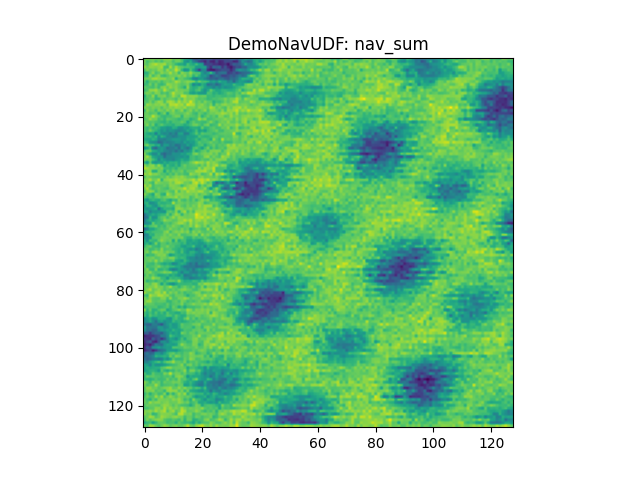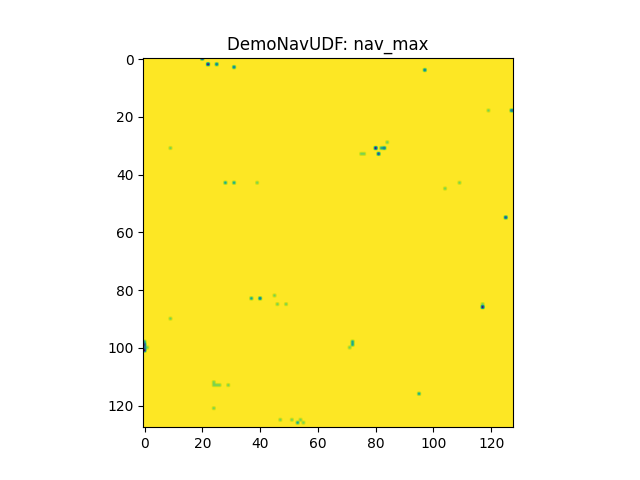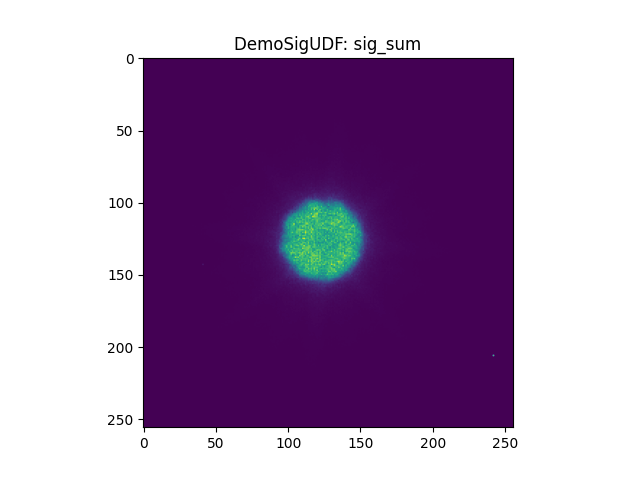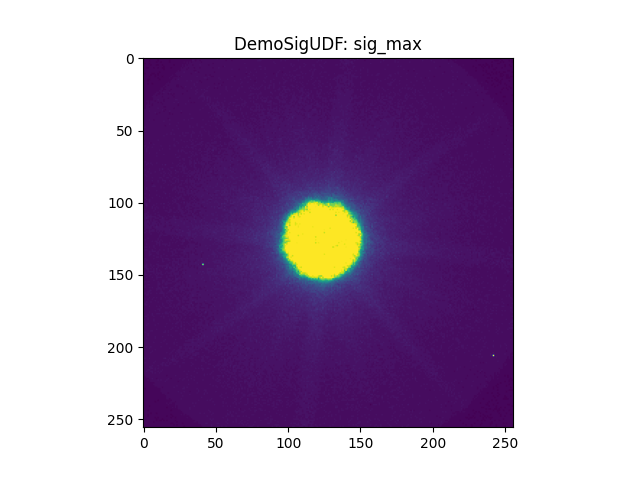# Live plotting for LiberTEM UDFs

This example demonstrates the live plotting feature of LiberTEM that is introduced in release 0.7.0. It can update a plot with new results while LiberTEM processing is running.

This notebook requires additional packages bqplot, bqplot_image_gl and ipywidgets. See also https://ipywidgets.readthedocs.io/en/stable/user_install.html#installing-in-classic-jupyter-notebook in case the plots don’t show.

:

%matplotlib nbagg

:

import os
import matplotlib.pyplot as plt
import matplotlib.colors
import ipywidgets
import IPython
import numpy as np

import libertem.api as lt
from libertem.udf import UDF
from libertem.udf.raw import PickUDF
from libertem.viz.mpl import MPLLive2DPlot
from libertem.viz.bqp import BQLive2DPlot


## Plot classes

Currently, LiberTEM implements three back-ends for live plotting:

• libertem.viz.mpl.MPLLive2DPlot with a matplotlib back-end. It is the default since matplotlib is the most popular and mature plotting package for Python. It requires about 100-200 ms to display or update a plot, which only allows a few updates per second.

• libertem.viz.bqp.BQLive2DPlot with a bqplot_image_gl back-end. It is much faster than matplotlib and can easily keep up with the full update rate of LiberTEM for a smoother and more responsive display.

• libertem.viz.gms.GMSLive2DPlot for plotting within Digital Micrograph, Gatan Microscopy Suite. This only works using the Python scripting of recent GMS releases and is not demonstrated here.

Additional plotting back-ends can be implemented by deriving from libertem.viz.base.Live2DPlot.

Here we change the default class for live plotting to BQLive2DPlot and then change it back right away by assigning to the plot_class property of the LiberTEM Context object.

:

# We set the Context to use BQLive2DPlot for live plotting
ctx = lt.Context(plot_class=BQLive2DPlot)

# We change it right back to the default MPLLive2DPlot
ctx.plot_class = MPLLive2DPlot

:

data_base_path = os.environ.get("TESTDATA_BASE_PATH", "/home/alex/Data/")
ds = ctx.load("auto", path=os.path.join(data_base_path, "20200518 165148/default.hdr"))

# This internal method is used here to artificially create more partitions than necessary
# for better demonstration of live plotting on machines with few cores.
# This reduces performance, should NOT be used in production and can change without
# notice in future releases.
ds.set_num_cores(32)


## Demo UDFs

We implement three simple user-defined functions (UDFs) to demonstrate the various features and options for live plotting. They calculate a map of the navigation space with sum and maximum, a map of the signal space with sum and maximum, and a global maximum.

:

class DemoNavUDF(UDF):
def get_result_buffers(self):
return {
'nav_sum': self.buffer(kind='nav', dtype='float32'),
'nav_max': self.buffer(kind='nav', dtype='float32'),
}

def process_frame(self, frame):
self.results.nav_sum[:] = np.sum(frame)
self.results.nav_max[:] = np.max(frame)

class DemoSigUDF(UDF):
def get_result_buffers(self):
return {
'sig_sum': self.buffer(kind='sig', dtype='float32'),
'sig_max': self.buffer(kind='sig', dtype='float32'),
}

def process_frame(self, frame):
self.results.sig_sum += frame
np.maximum(self.results.sig_max, frame, out=self.results.sig_max)

def merge(self, dest, src):
dest.sig_sum += src.sig_sum
np.maximum(dest.sig_max, src.sig_max, out=dest.sig_max)

class DemoSingleUDF(UDF):
def get_result_buffers(self):
return {
'maximum': self.buffer(kind='single', dtype='float32'),
}

def process_frame(self, frame):
self.results.maximum[:] = np.maximum(self.results.maximum, np.max(frame))

def merge(self, dest, src):
dest.maximum[:] = np.maximum(dest.maximum, src.maximum)


The UDFs are instantiated and stored in a list for subsequent use.

:

udfs = [DemoNavUDF(), DemoSigUDF(), DemoSingleUDF()]


## Plot all plottable channels

Note how Context.run_udf() can execute several UDFs in one pass since release 0.7.0 by passing a list or tuple of UDFs.

By setting plots=True you can plot all channels that have a 2D shape after applying np.squeeze. The DemoSingleUDF is not plotted since its only channel maximum is a single value. This triggers a warning.

:

res = ctx.run_udf(dataset=ds, udf=udfs, plots=True)## Select specific channels

We can specify which channels should be plotted by passing a nested list with channel names for each of the UDFs as the plots parameter.

:

res = ctx.run_udf(dataset=ds, udf=udfs, plots=[['nav_sum'], ['sig_sum', 'sig_max']])